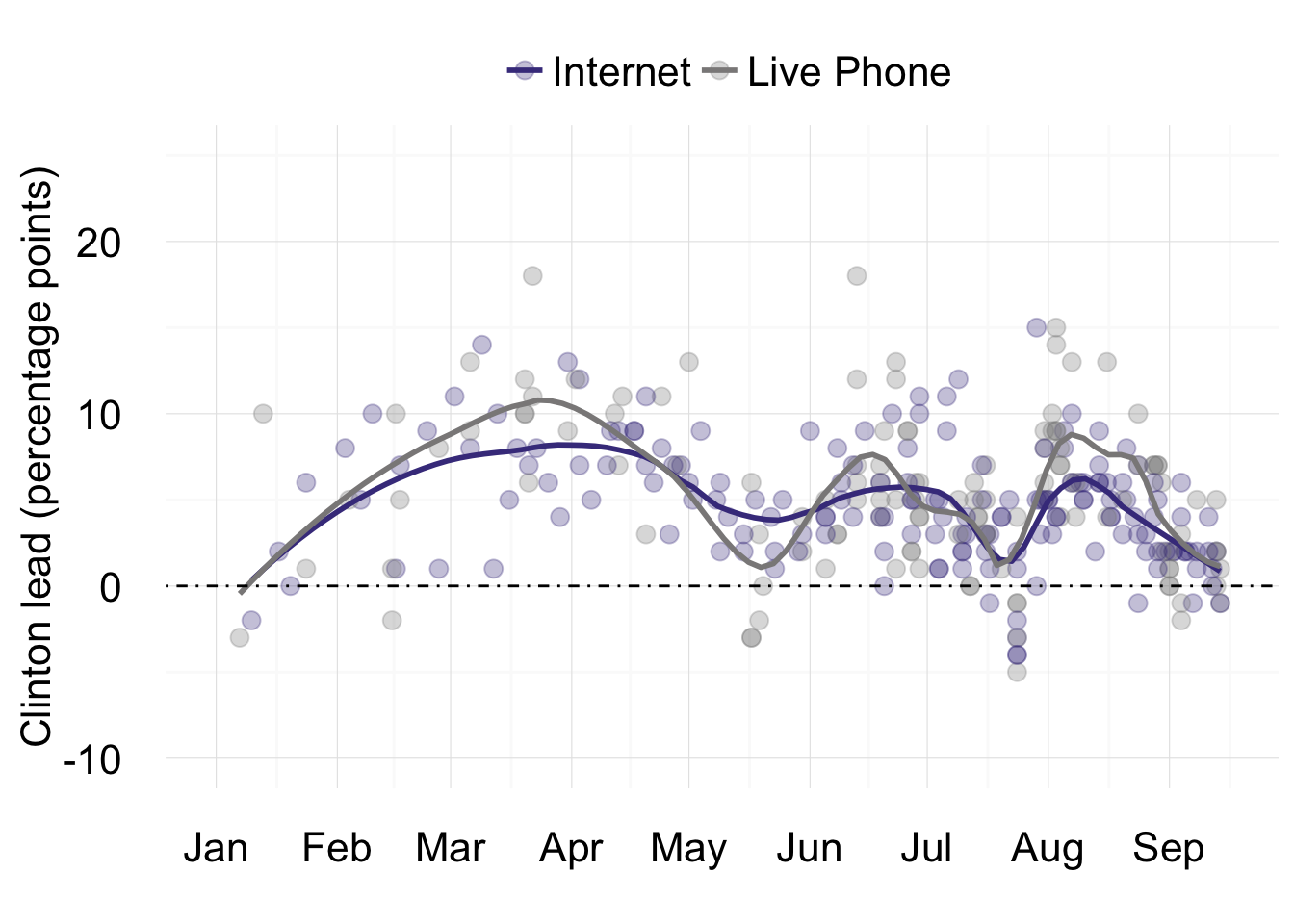# Why “Shy Trumpers” Probably Won’t Decide The Election

R code for the analysis supporting this September 16, 2016 BuzzFeed News article on the possibility of a hidden army of Trump supporters, embarrassed to reveal their voting preference to pollsters, being the deciding factor in the 2016 presidential election. Supporting files are in this GitHub repository.

## Data preparation

The following code loads data on presidential election polls from Huffington Post Pollster, then filters to include only independent online and live telephone polls of registered or likely voters, where the sample size was declared. All of the polls in the filtered data collected responses from more than 500 people.

``````# load required packages
library(dplyr)

names(polls) <- c("Pollster","StartDate","EndDate","EntryDate/Time(ET)","NumberofObservations","Population","Mode","Trump","Clinton","Other","Undecided","PollsterURL","SourceURL","Partisan","Affiliation","QuestionText","QuestionIteration")

polls <- polls %>%

# filter for independent polls of likely or registered voters from entire electorate, where sample size is declared
polls_edit <- polls %>%
filter(Population=="Likely Voters" | Population=="Registered Voters",
Mode=="Internet" | Mode == "Live Phone",
Affiliation == "None" | Affiliation == "Nonpartisan",
!is.na(NumberofObservations))``````

## Charts

The code that follows makes charts showing results for polls with end dates from January 1, 2016 onwards. The lines are drawn by loess regression using the `geom_smooth` function in the ggplot2 package, setting the `span` to 0.3.

### The presidential horse race, in online vs. telephone polls.

``````# load required package
library(ggplot2)

# horse race
geom_point(aes(color=Mode), size=3, alpha=0.3) +
geom_smooth(aes(color=Mode), se=F, span=0.3) +
scale_color_manual(values = c("slateblue4","snow4")) +
xlab("") +
theme_minimal() +
scale_x_date(limits = c(as.Date("2016-01-01"), Sys.Date()),
date_breaks = "1 month", date_labels = "%b") +
scale_y_continuous(limits = c(-10,25)) +
theme(text=element_text(size=20),
axis.title = element_text(size=16),
legend.position = "top",
legend.title = element_blank()) +
geom_hline(yintercept=0, linetype="dotdash")### Trump’s support has been slightly stronger in live polls than in those run online.

``````# trump support
trump <- ggplot(polls_edit, aes(x=EndDate, y=Trump, group=Mode)) +
geom_point(aes(color=Mode), size=3, alpha=0.3) +
geom_smooth(aes(color=Mode), se=F, span=0.3) +
scale_color_manual(values = c("slateblue4","snow4")) +
xlab("") +
ylab("Trump support (%)") +
theme_minimal() +
scale_x_date(limits = c(as.Date("2016-01-01"), Sys.Date()),
date_breaks = "1 month", date_labels = "%b") +
scale_y_continuous(limits = c(25,60)) +
theme(text=element_text(size=20),
axis.title = element_text(size=16),
legend.position = "top",
legend.title = element_blank())

plot(trump)``````